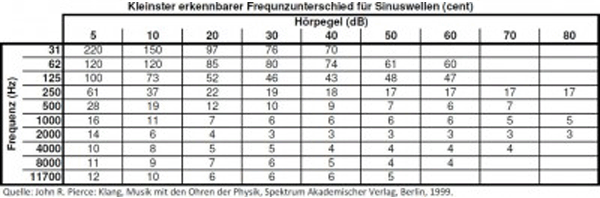Deutsche VersionConversion  of  Intervals  −  ¢ = cent

Frequency ratio to cents and cents to frequency  ratio

Change of pitch with change of temperature

1 hertz = 1 Hz = cps = cycles per second
The unit most commonly used to measure intervals is called cent, from Latin centum,
meaning "one hundred". It stands for one hundredth of an equal-tempered semitone.
In other words, one octave consists of 1200 cents.

 cents | Frequency ratio f2 / f1 | ↓ | ↓ | Frequency ratio f2 / f1 | cents

Fill out the gray boxes and click at the calculation bar.

How many cents are in a hertz?
There is no conversion from Hz to cents and vice versa.

Scroll down to the bottom: "Table of Cent Difference".

Statement: Cent is a logarithmic unit of measure of an interval, and that is a dimensionless "frequency ratio" of f2 / f1.

Calculation: Intervals (cents) and Frequency (Hz) as Excel Program (xls)

Different Thirds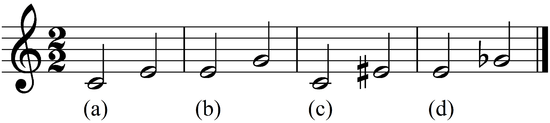Cent value-determination of an interval

 Frequency f1 Hz (hertz) Frequency f2 Hz (hertz) ↓ Interval cents Ratio f2 / f1

Instead of the frequencies you can take the fraction numbers − e.g. 4/5 of the interval major third.

 Formula for converting the interval frequency ratio f2 / f1 to cents (c or ¢). ¢ or c = 1200 × log2 (f2 / f1) log 2 = 0.301029995
This formula employs a log 2, or logarithm base 2 function. This formula can also be
written using a log 10 function, available on most scientific calculators via the log button:
 c = 1200 × 3.322038403 log10 (f2 / f1) 1/log 2 = 1/0.301029995 = 3.322038403 The formula expressed using log10 rather than log 2.
3.322038403 is a conversion factor that converts base 2 logarithms to base 10 logarithms.
1 Cent = 2(1/1200) = 1.0005777895065548592967925757932
One cent is thus the number that multiplied by itself 1200 times results in the number 2.
The cent is an interval which is calculated from the interval frequency ratio as follows:
(In of the interval frequency ratio / ln 2)×1200 = cents value of the interval.
An interval of a halftone is equivalent to: 2(1/12) = 1,0594630943592952645618252949463.
That is: [ln (2(1/12)) / ln (2)]×1200 cent = 100 cent.

 The Pythagorean comma is the frequency ratio (3 / 2)12 / 27 = 312 / 219 = 531441 / 524288 = 1.0136432647705078125. The resulting is converted to 23.460010384649013 cent. Twelve perfect fifths (3 / 2) reveals 8423.46 cents and seven octaves, however, reveals only 8400 cents.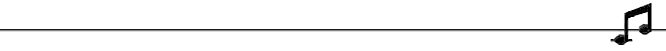Conversions of Semitone Intervals
An interval is the difference between two pitches or the
distance between two frequencies in terms of semitones
.

 Enter any two known values and press "calculate" to solve for the other. Please, enter only two values.

 Frequency f1 Hz Frequency f2 Hz Number of semitonesFrequency calculation for different octave intervals

 Initial tone f Hz Step of  steps ↓ New frequency f HzChange of pitch with change of temperatureChanging of the frequency about a cent value

 Initial tone f Hz Change of pitch J cents ↓ New frequency f HzFrequency to musical note converter

Find out what note a given frequency is. English system.

 Frequency f Hz ↓ Musical note Offset cents
 Formulas: f = 440 × 2(−58/12) × (2(1/12))n and f = 440 × 2((n−58)/12)   The original source program of Iain W. Bird is still faulty: http://www.birdsoft.demon.co.uk/music/notecalc.htm   The frequency of 440 Hz is the concert pitch note A4. If someone tells you different, this person is in error; see also:The frequency as semitone distance from A4 = 440 Hz

 For a note that lies n semitones higher (or −n semitones lower) from A4, the frequency is fn = 2n/12 × 440 Hz. Conversely, one can obtain n, the number of semitones from A4, from: n = 12 × log2 (fn / 440 Hz).

 To use the calculator, simply enter a value. The calculator works in both directions of the ↔ sign.

 Semitone n as distance from A4 Semitones ↔ Frequency fn - Reference: 440 Hz  Hz n = 12*(log (fn/440) / log(2))                                        fn = 2^(n/12)*440Masterclock calculator (clock rate)

 To use the calculator, simply enter a value. The calculator works in both directions of the ↔ sign.

 Reference wordclock 48.0 kHz  Piano tuning f Hz ↔ Reference frequency 440 Hz  Studio wordclock fs kHz

 Reference wordclock 44.1 kHz  Piano tuning f  Hz ↔ Reference frequency 440 Hz  Studio wordclock fs kHzCalculator with reference frequency

 Reference wordclock kHz Reference frequency Hz Piano tuning f Hz ↓ Studio wordclock fs kHz Interval deviation cent

For downward tuning the reference frequency and piano tuning can be changed.

100 cent is equivalent to a semitone (halftone).Octave division in 12-tone equal temperament TET

 Interval Frequency ratio cents Unison 1.000000 : 1 0 Semitone or minor second 1.059463 : 1 100 Whole tone or major second 1.122462 : 1 200 Minor third 1.189207 : 1 300 Major third 1.259921 : 1 400 Perfect fourth 1.334840 : 1 500 Augmented fourth/Diminished fifth 1.414214 : 1 600 Perfect fifth 1.498307 : 1 700 Minor sixth 1.587401 : 1 800 Major sixth 1.681793 : 1 900 Minor seventh 1.781797 : 1 1000 Major seventh 1.887749 : 1 1100 Octave 2.000000 : 1 1200

Some like to tell us that calling a tempered fifth "perfect"
is a misnomer and perfect intervals are only proper fractions.

KeyboardIn the following table are for the most popular pure dyads up to the octave - the frequency ratio is themeasure of consonance and the sound sensation of most people.

 Dyads Frequencyratio Consonancevalue Sensation of sound minor second 16:15 15.49 very dissonant major second 9:8 8.49 dissonant minor third 6:5 5.48 consonant ("minor") major third 5:4 4.47 consonant ("major") forth 4:3 3.46 consonant tritonus 45:32 37.95 very dissonant fifth 3:2 2.45 very consonant ("neutral") minor sixth 8:5 6.32 consonant ("minor") major sixth 5:3 3.87 consonant ("major") minor seventh 16:9 12.00 dissonant major seventh 15:8 10.95 dissonant octave 2:1 1.41 very consonant ("neutral")

 Name Exact value in 12-TET Decimal value Just intonation interval Percent difference Unison 1 1.000000 1 = 1.000000 0.00% Minor second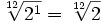1.059463 16/15 = 1.066667 −0.68% Major second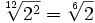1.122462 9/8 = 1.125000 −0.23% Minor third1.189207 6/5 = 1.200000 −0.91% Major third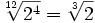1.259921 5/4 = 1.250000 +0.79% Perfect fourth1.334840 4/3 = 1.333333 +0.11% Diminished fifth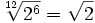1.414214 7/5 = 1.400000 +1.02% Perfect fifth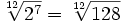1.498307 3/2 = 1.500000 −0.11% Minor sixth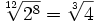1.587401 8/5 = 1.600000 −0.79% Major sixth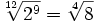1.681793 5/3 = 1.666667 +0.90% Minor seventh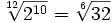1.781797 16/9 = 1.777778 +0.23% Major seventh1.887749 15/8 = 1.875000 +0.68% Octave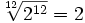2.000000 2/1 = 2.000000 0.00%

 TET 12 - equal temperament   semitone    (1/2 tone) has the frequency ratio of 12√2 = 21/12 = 1.0594630943592952645618252949463 TET 24 - equal temperament quarter tone (1/4 tone) has the frequency ratio of 24√2 = 21/24 = 1.0293022366434920287823718007739 TET 48 - equal temperament eighth tone   (1/8 tone) has the frequency ratio of 48√2 = 21/48 = 1.0145453349375236414538678576629

 Question: How can I convert cents to Hz? Answer: You cannot convert cents to hertz, because cents are not a frequency. cents are the measurement between intervals, that is a "frequency ratio" f2/f1.

Here's a Table of Cents Difference for some frequencies close around 440 Hz:

 Frequency Difference 435 Hz −19.78 cents 436 Hz −15.81 cents 437 Hz −11.84 cents 438 Hz −7.89 cents 439 Hz −3.94 cents 440 Hz ±0 cent 441 Hz +3.93 cents 442 Hz +7.85 cents 443 Hz +11.76 cents 444 Hz +15.67 cents 445 Hz +19.56 cents

So, the conversion factor 4 cents / Hz is valid for the purposes of tuning as an exception only very close around 440 Hz.

There is no conversion from Hz to cents and vice versa.
Statement: Cent is a logarithmic unit of measure of an interval, and that is a dimensionless "frequency ratio" of f2 / f1.

Smallest recognizable frequency difference for pure tones at different listening levels